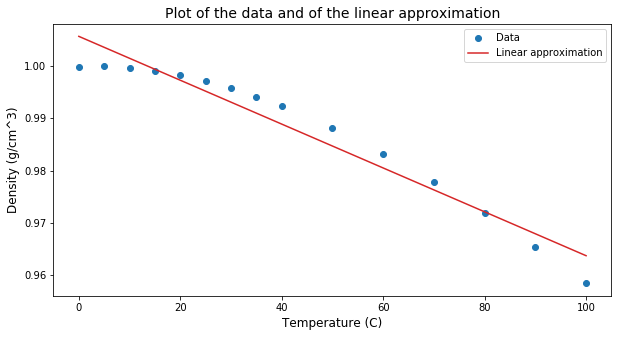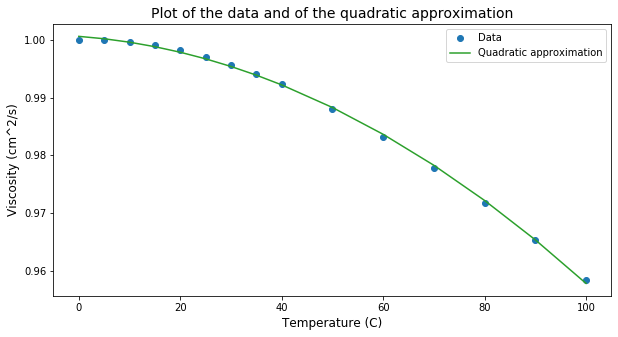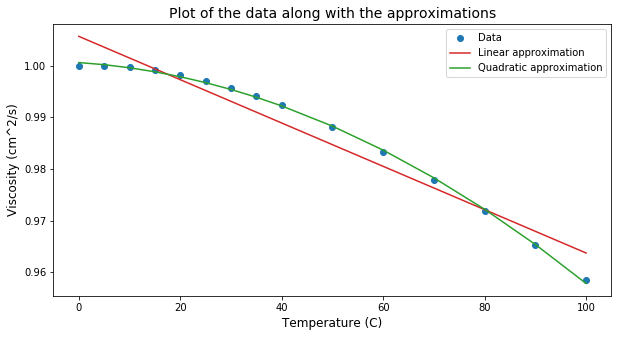# Low-Dimensional Fittings¶

We use the classical least-square method to fit low degree polynomials to the data.

See e.g. Golub and Van Loan (1996) and Trefethen and Bau III (1997) for details on the least-square method.

## Importing the libraries¶

In :
import csv
import numpy as np
import matplotlib.pyplot as plt


This time we use the csv library to read the data from file and use numpy to work with the data.

We first load the data into a python list with csv.

In :
file = open('water.csv',"r")
water_csv = list(csv.reader(file, delimiter=","))
water_csv

Out:
[['temp', 'density', 'viscosity'],
['Temperature (C)', 'Density (g/cm^3)', 'Viscosity (cm^2/s)'],
['0', '0.9999', '0.01787'],
['5', '1', '1.514'],
['10', '0.9997', '1.304'],
['15', '0.9991', '1.138'],
['20', '0.9982', '1.004'],
['25', '0.9971', '0.894'],
['30', '0.9957', '0.802'],
['35', '0.9941', '0.725'],
['40', '0.9923', '0.659'],
['50', '0.9881', '0.554'],
['60', '0.9832', '0.475'],
['70', '0.9778', '0.414'],
['80', '0.9718', '0.366'],
['90', '0.9653', '0.327'],
['100', '0.9584', '0.295']]

### Header and datapoints¶

We separate the header from the datapoints and have the datapoints put into a numpy array as follows.

In :
header = dict([(water_csv[i],water_csv[i]) for i in range(3)])

{'temp': 'Temperature (C)', 'density': 'Density (g/cm^3)', 'viscosity': 'Viscosity (cm^2/s)'}

In :
datapoints = np.array(water_csv[2:]).astype("float")
print(datapoints)

[[0.000e+00 9.999e-01 1.787e-02]
[5.000e+00 1.000e+00 1.514e+00]
[1.000e+01 9.997e-01 1.304e+00]
[1.500e+01 9.991e-01 1.138e+00]
[2.000e+01 9.982e-01 1.004e+00]
[2.500e+01 9.971e-01 8.940e-01]
[3.000e+01 9.957e-01 8.020e-01]
[3.500e+01 9.941e-01 7.250e-01]
[4.000e+01 9.923e-01 6.590e-01]
[5.000e+01 9.881e-01 5.540e-01]
[6.000e+01 9.832e-01 4.750e-01]
[7.000e+01 9.778e-01 4.140e-01]
[8.000e+01 9.718e-01 3.660e-01]
[9.000e+01 9.653e-01 3.270e-01]
[1.000e+02 9.584e-01 2.950e-01]]


## Linear approximation¶

Given a set of temperature and density data in the form $(T_j, \rho_j)$, we look for a linear relation $\rho^{(1)}(T) = c + m T$ which is the "best fit" for the data.

One way to approach this problem is to interpret the best fit as minimizing the sum of squares of the residuals. The residual for each $j$ measurement is

$$r_j = \rho_j - \rho^{(1)}(T_j),$$

and the sum of squares of the residuals is

$$\min_{c,m\in \mathbb{R}} \sum_j (\rho_j - \rho^{(1)}(T))^2.$$

### Matrix form¶

This can be written in matrix form as

$$\displaystyle \min_{\mathbf{u}\in \mathbb{R}^2} \|A\mathbf{u} - \mathbf{f}\|_2^2,$$

where $\|\cdot\|_2$ is the Euclidian norm of a vector and

$$A = \left[ \begin{matrix} T_1 & 1 \\ \vdots & 1 \\ T_n & 1 \end{matrix}\right], \qquad \mathbf{u} = \left( \begin{matrix} m \\ c \end{matrix}\right), \qquad \mathbf{f} = \left( \begin{matrix} \rho_1 \\ \vdots \\ \rho_n \end{matrix} \right).$$

The matrix $A$ is a simple Vandermonde type matrix obtained from the temperature data, $\mathbf{u}$ is the unknown vector with the desired coefficients for the approximation, and $\mathbf{f}$ is the vector with the density measurements.

### The Vandermonde matrix¶

We use the numpy function numpy.vstack() to build the Vandermonde matrix $A$.

In :
T = datapoints[:,0]
A1 = np.vstack([T,np.ones(len(T))]).T
print(A1)

[[  0.   1.]
[  5.   1.]
[ 10.   1.]
[ 15.   1.]
[ 20.   1.]
[ 25.   1.]
[ 30.   1.]
[ 35.   1.]
[ 40.   1.]
[ 50.   1.]
[ 60.   1.]
[ 70.   1.]
[ 80.   1.]
[ 90.   1.]
[100.   1.]]


### The density measurements¶

The density data is the second column of the data array.

In :
f = datapoints[:,1]
print(f)

[0.9999 1.     0.9997 0.9991 0.9982 0.9971 0.9957 0.9941 0.9923 0.9881
0.9832 0.9778 0.9718 0.9653 0.9584]


### Solution of the least-square problem for the linear approximation¶

We use the numpy function numpy.linagl.lstsq() to solve the least-square problem.

In :
m, d = np.linalg.lstsq(A1, f, rcond=None)
print(m,d)

-0.00041965346534652876 1.0056721122112207


### Visualizing the result¶

Now we plot the linear approximation along with the data to visualize the quality of the approximation

In :
plt.figure(figsize=(10,5))
plt.plot(T, f, 'o', label='Data', color='tab:blue')
plt.plot(T, m*T + d, 'b', label='Linear approximation', color='tab:red')
plt.title('Plot of the data and of the linear approximation', fontsize=14)
plt.legend()
plt.show()## Second-degree approximation¶

One can see from the plot above that the first-degree approximation doesn't seem to be a very good approximation. We look, now, for a second-degree approximation.

### The least-square problem¶

For the second-degree approximation, we look for a second-degree polynomial $\rho^{(2)}(T) = aT^2 + bT + c$ that best approximates the data in the sense of minimizing the sum of the square of the residuals

$$r_j = \rho_j - \rho^{(2)}(T_j)$$

### Matrix form¶

This can be written in matrix form as

$$\displaystyle \min_{\mathbf{u}\in \mathbb{R}^2} \|A\mathbf{u} - \mathbf{f}\|_2^2,$$

where $\mathbf{f}$ is as before but the Vandermonde matrix and the vector of unknowns take the form

$$A = \left[ \begin{matrix} T_1^2 & T_1 & 1 \\ \vdots & 1 \\ T_n^2 & T_n & 1 \end{matrix}\right], \qquad \mathbf{u} = \left( \begin{matrix} a \\ b \\ c \end{matrix}\right).$$

### Vandermonde matrix¶

In this case, the Vandermonde matrix is

In :
A2 = np.vstack([T**2, T,np.ones(len(T))]).T
print(A2)

[[0.000e+00 0.000e+00 1.000e+00]
[2.500e+01 5.000e+00 1.000e+00]
[1.000e+02 1.000e+01 1.000e+00]
[2.250e+02 1.500e+01 1.000e+00]
[4.000e+02 2.000e+01 1.000e+00]
[6.250e+02 2.500e+01 1.000e+00]
[9.000e+02 3.000e+01 1.000e+00]
[1.225e+03 3.500e+01 1.000e+00]
[1.600e+03 4.000e+01 1.000e+00]
[2.500e+03 5.000e+01 1.000e+00]
[3.600e+03 6.000e+01 1.000e+00]
[4.900e+03 7.000e+01 1.000e+00]
[6.400e+03 8.000e+01 1.000e+00]
[8.100e+03 9.000e+01 1.000e+00]
[1.000e+04 1.000e+02 1.000e+00]]


### Solution¶

In :
a, b, c = np.linalg.lstsq(A2, f, rcond=None)
print(a,b,c)

-3.6295100056677867e-06 -6.496768558464583e-05 1.0005991832098982


### Visualizing the result¶

In :
plt.figure(figsize=(10,5))
plt.plot(T, f, 'o', label='Data', color='tab:blue')
plt.plot(T, a*T**2 + b*T + c, 'r', label='Quadratic approximation', color='tab:green')
plt.title('Plot of the data and of the quadratic approximation', fontsize=14)
plt.legend()
plt.show()This seems much better

## Comparing the two approximations¶

### Visual comparison¶

Visually, the second-degree approximation is way better.

In :
plt.figure(figsize=(10,5))
plt.plot(T, f, 'o', label='Data', color='tab:blue')
plt.plot(T, m*T + d, 'b', label='Linear approximation', color='tab:red')
plt.plot(T, a*T**2 + b*T + c, 'r', label='Quadratic approximation', color='tab:green')
plt.title('Plot of the data along with the approximations', fontsize=14)
plt.legend()
plt.show()### Comparing the quadratic error¶

The quadratic error is the sum of the square of the residual errors for each measurement, i.e.

$$\Delta = \sum_j r_j^2 = \|A\mathbf{u} - \mathbf{f}\|_2^2,$$

for the best approximation obtained. But this error is not normalized by the number of measurements. This is achieved with the mean quadratic error:

$$E = \frac{1}{N} \sum_j r_j^2.$$
In :
N = len(f)
print(f'Number of measurements: {N}\n')
print(f'Quadratic error for the linear approximation: {np.linalg.lstsq(A1, f, rcond=None):.2e}')
print(f'Quadratic error for the quadratic approximation: {np.linalg.lstsq(A2, f, rcond=None):.2e}\n')
print(f'Mean quadratic error for the linear approximation: {np.linalg.lstsq(A1, f, rcond=None)/N:.2e}')
print(f'Mean quadratic error for the quadratic approximation: {np.linalg.lstsq(A2, f, rcond=None)/N:.2e}')

Number of measurements: 15

Quadratic error for the linear approximation: 1.38e-04
Quadratic error for the quadratic approximation: 1.99e-06

Mean quadratic error for the linear approximation: 9.22e-06
Mean quadratic error for the quadratic approximation: 1.33e-07